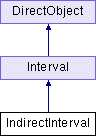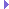IndirectInterval Class Reference
Inheritance diagram for IndirectInterval:## Public Member Functions

def __init__ (self, interval, startT=0, endT=None, playRate=1, duration=None, blendType='noBlend', name=None)

def privFinalize (self)

def privInitialize (self, t)

def privInstant (self)

def privInterrupt (self)

def privReverseFinalize (self)

def privReverseInitialize (self, t)

def privReverseInstant (self)

def privStep (self, t)Public Member Functions inherited from Interval
def __init__ (self, name, duration, openEnded=1)

def __repr__ (self, indent=0)

def clearToInitial (self)

def finish (self)

def getDoneEvent (self)

def getDuration (self)

def getLoop (self)

def getName (self)

def getOpenEnded (self)

def getPlayRate (self)

def getState (self)

def getT (self)

def intervalDone (self)

def isPaused (self)

def isPlaying (self)

def isStopped (self)

def loop (self, startT=0.0, endT=-1.0, playRate=1.0)

def pause (self)

def popupControls (self, tl=None)

def privDoEvent (self, t, event)

def privPostEvent (self)

def resume (self, startT=None)

def resumeUntil (self, endT)

def setDoneEvent (self, event)

def setLoop (self, loop=1)

def setPlayRate (self, playRate)

def setT (self, t)

def setupPlay (self, startT, endT, playRate, doLoop)

def setupResume (self)

def start (self, startT=0.0, endT=-1.0, playRate=1.0)

def stepPlay (self)Public Member Functions inherited from DirectObject
def __init__ (self)

def accept (self, event, method, extraArgs=[])

def acceptOnce (self, event, method, extraArgs=[])

def addTask (self, *args, **kwargs)

def detectLeaks (self)

def doMethodLater (self, *args, **kwargs)

def getAllAccepting (self)

def ignore (self, event)

def ignoreAll (self)

def isAccepting (self, event)

def isIgnoring (self, event)

def removeAllTasks (self)

def removeTask (self, taskOrName)

## Public Attributes

blendType

currT

deltaT

endAtEnd

endT

interval

startAtStart

startT

statePublic Attributes inherited from Interval
currT

doneEvent

duration

es

name

openEnded

pname

pstats

setTHooks

state

## Static Public Attributes

int indirectIntervalNum = 1

notify = directNotify.newCategory('IndirectInterval')Static Public Attributes inherited from Interval
notify = directNotify.newCategory("Interval")

int playbackCounter = 0Static Public Attributes inherited from DirectObject
def accept_once = acceptOnce

def add_task = addTask

def detect_leaks = detectLeaks

def do_method_later = doMethodLater

def get_all_accepting = getAllAccepting

def ignore_all = ignoreAll

def is_accepting = isAccepting

def is_ignoring = isIgnoring

def remove_all_tasks = removeAllTasks

def remove_task = removeTask

## Additional Inherited MembersProperties inherited from Interval
done_event = property(getDoneEvent, setDoneEvent)

open_ended = property(getOpenEnded)

play_rate = property(getPlayRate, setPlayRate)

stopped = property(isStopped)

t = property(getT, setT)

## Detailed Description

```This class can be used to play samples of another interval, so
that only a subset of the interval is played, or the time is
compressed, or some such nonsense.

It keeps a reference to the interval itself and repeatedly calls
setT() on it, rather than actually starting the interval or
copying its members like Sequence() or Parallel().  This means two
IndirectIntervals that operate on the same nested interval may
have some interaction that you should be aware of.
```

## ◆ __init__()

 def __init__ ( self, interval, startT = `0`, endT = `None`, playRate = `1`, duration = `None`, blendType = `'noBlend'`, name = `None` )

## ◆ privFinalize()

 def privFinalize ( self )

Reimplemented from Interval.

## ◆ privInitialize()

 def privInitialize ( self, t )

Reimplemented from Interval.

## ◆ privInstant()

 def privInstant ( self )

Reimplemented from Interval.

## ◆ privInterrupt()

 def privInterrupt ( self )

Reimplemented from Interval.

## ◆ privReverseFinalize()

 def privReverseFinalize ( self )

Reimplemented from Interval.

## ◆ privReverseInitialize()

 def privReverseInitialize ( self, t )

Reimplemented from Interval.

## ◆ privReverseInstant()

 def privReverseInstant ( self )

Reimplemented from Interval.

## ◆ privStep()

 def privStep ( self, t )

Reimplemented from Interval.

## ◆ blendType

 blendType

 currT

 deltaT

## ◆ endAtEnd

 endAtEnd

 endT

## ◆ indirectIntervalNum

 int indirectIntervalNum = 1
static

## ◆ interval

 interval

## ◆ notify

 notify = directNotify.newCategory('IndirectInterval')
static

## ◆ startAtStart

 startAtStart

 startT

 state